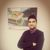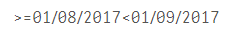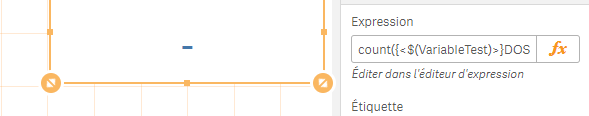Announcements
The way to achieve your own success is the willingness to help somebody else. Go for it!
cancel
Showing results for
Did you mean:Specialist

## Set Analysis Month -1

Hello I have this set analysis like Month to date :

DateCal=,Year=,Month=,Quarter=,

DateCal={">=\$(=MonthStart(max(DateCal)))<=\$(=date(max(DateCal)))"}

I would like now to do Month to date -1 (if i select April  2017 i will have March 2017 )

Thanks for help

1 Solution

Accepted SolutionsSpecialist
Author

Hello,

I find the problem i need to add year=,month = in the variable :

Year=,Month=,DateCal = {"\$(='>=' & MonthStart(Max(DateCal),-1) & '<' & MonthStart(Max(DateCal)))"}

14 RepliesMVP

Month=,Quarter=,

DateCal={">=\$(=MonthStart(max(DateCal),-1))<\$(=MonthStart(max(DateCal)))"}MVP

It should be sthing like:

DateCal={">=\$(=MonthStart(max(DateCal),-1))<\$(=MonthStart(max(DateCal)))"}

or

DateCal={">=\$(=MonthStart(max(DateCal),-1))<=\$(=MonthEnd(max(DateCal),-1))"}Specialist
Author

Hello Stefan,

I used this but it doesn't workMVP

the try :MVP

What date range and format do you see when you add this to a KPI object?

='>=' & MonthStart(Max(DateCal),-1) & '<' & MonthStart(Max(DateCal))

Do you see the >=03/01/2017<04/01/2017 when you select April? and also in the format of your field DateCal?Specialist
Author

Hello,

Thanks for all,

I don't know why when i try this in text object :

='>=' & MonthStart(Max(DateCal),-1) & '<' & MonthStart(Max(DateCal))

I have the good result :But when i put it in variable and i use it in the set analysis like that :I have this :MVP

Try with this variable

DateCal = {"\$(='>=' & MonthStart(Max(DateCal),-1) & '<' & MonthStart(Max(DateCal)))"}Specialist
Author

I used this variable :

DateCal = {"\$(='>=' & MonthStart(Max(DateCal),-1) & '<' & MonthStart(Max(DateCal)))"}

and this set analysis and I have 0 :

count({<\$(var)>}Docs)MVP

Can you share an image of chart where you use this expression. Make sure to not label the expression... I would like to see how this get's expanded in the labelCommunity Browser